灰度变换_灰度变换增强 - CSDN

• 对于数字图像处理而言，...空间域处理方法主要是直接以图像中的像素操作为基础，它主要分为灰度变换和空间滤波两类。灰度变换是在图像的单个像素上操作，主要以对比度和阈值处理为目的。空间域处理可由下式表示： ...
        对于数字图像处理而言，一般包含着空间域处理和变换域处理两种形式。空间域处理方法主要是直接以图像中的像素操作为基础，它主要分为灰度变换和空间滤波两类。灰度变换是在图像的单个像素上操作，主要以对比度和阈值处理为目的。空间域处理可由下式表示：

g(x,y)=T[f(x,y)]

其中f(x,y)是输入图像，g(x,y)是处理后的图像，T是在点(x,y)的领域上定义的关于f的一种算子。

为了保证经过灰度变换后的输出图像在整体的外貌上，或者更准确地说在形态学上，与输入图像保持一致，灰度变换函数必须是严格单调递增函数。在图像处理中，灰度变换主要应用于图像的对比度改善。在灰度变换中，最为常用的三类基本函数为线性函数（反转和恒等变换）、对数函数（对数和反对数变换）以及幂律函数（n次幂和n次根变换）。恒等函数是最一般的情况，其输出图像灰度等于输入图像灰度的变换。

1、图像反转

图像反转的表达式：       s=L-1-r   。其中r表示处理前的灰度值，s表示处理后的灰度值。采取这种方式反转一副图像的灰度级，可得到等效的照片底片。特别适用于增强嵌入在一副图像的暗区域中的白色或者灰色细节，尤其是当黑色面积在尺寸上占主导地位时。

2、对数变换

对数变换的通用形式为：

s=c log(1+r)

其中c是一个常数，并假设r≥0，其变换曲线如下图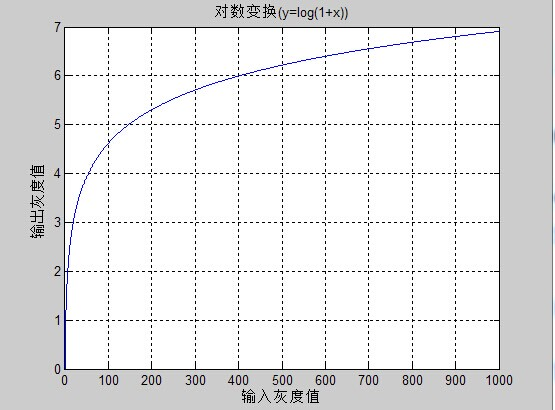对数变换将输入中范围较窄的低灰度值映射为输出中较宽范围的灰度值，相反，对高的输入灰度值也是如此。也就是说，该变换扩展图像的低灰度范围，同时压缩图像的高灰度范围。

3、幂律变换

幂律变换的基本表达式为：

其中c和γ为正常数。其一般表达式的图像为：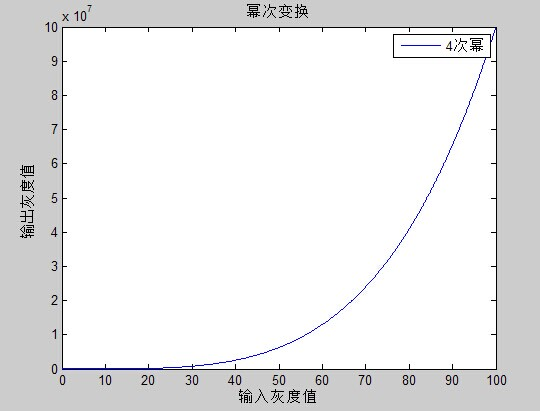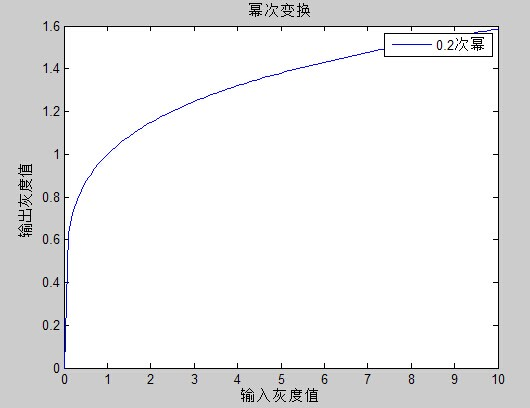对于γ<1，扩展低灰度范围，压缩高灰度范围；对于γ>1，压缩低灰度范围，扩展高灰度范围。

4、分段线性变换函数

对比度拉伸是最简单的分段线性函数。其一般的函数图像为：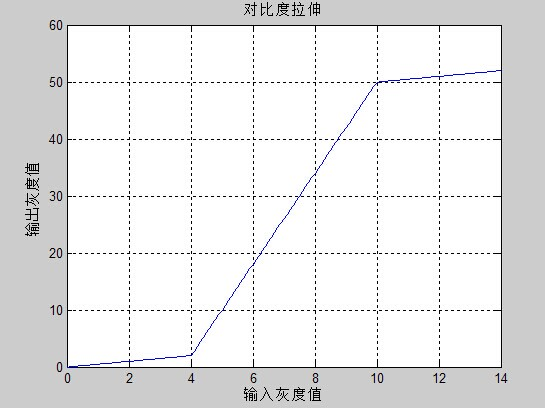展开全文• 1 灰度变换简介 2 线性灰度变换­—图像反转 3 非线性灰度变换 3.1 对数变换 3.2 伽马变换 参考资料 1 灰度变换简介 灰度变换是图像增强的一种重要手段，用于改善图像显示效果，属于空间域处理方法，它可以使...目录

1 灰度变换简介

2 线性灰度变换­—图像反转

3 非线性灰度变换

3.1 对数变换

3.2 伽马变换

参考资料

1 灰度变换简介

灰度变换是图像增强的一种重要手段，用于改善图像显示效果，属于空间域处理方法，它可以使图像动态范围加大，使图像对比度扩展，图像更加清晰，特征更加明显。灰度变换其实质就是按一定的规则修改图像每一个像素的灰度，从而改变图像的灰度范围。常见的灰度变换图像反转，对数变换和伽马变换等。其具体分类如下图所示：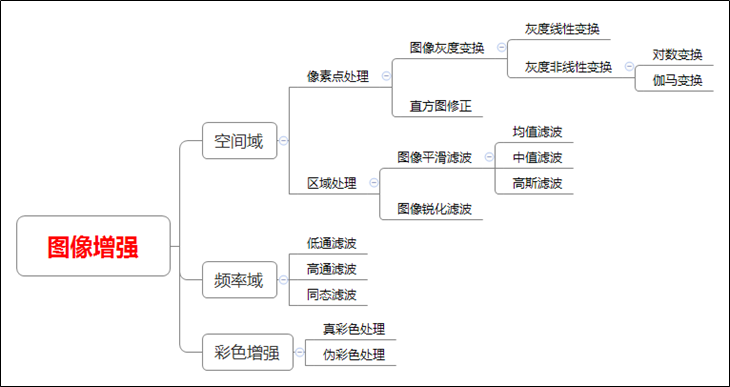2 线性灰度变换­—图像反转

灰度线性变换最常见的就是图像反转，在灰度图像灰度级范围中，其反转的公式如下所示：

其中，表示原始图像的灰度级，表示变换后的灰度级。

下图所示为图像反转的例子，原图像是数字乳房X射线照片，其中显示有一小块病变，通过图像反转就很容易看到病变区域。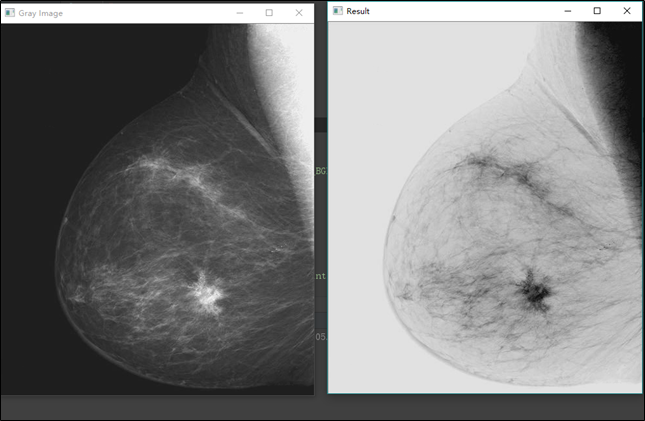代码如下所示：

# -*- coding: utf-8 -*-
import cv2
import numpy as np
import matplotlib.pyplot as plt

#读取原始图像

#图像灰度转换
grayImage = cv2.cvtColor(img, cv2.COLOR_BGR2GRAY)

#获取图像高度和宽度
height = grayImage.shape
width = grayImage.shape

#创建一幅图像
result = np.zeros((height, width), np.uint8)

#图像灰度反色变换 s=255-r
for i in range(height):
for j in range(width):
gray = 255 - grayImage[i,j]
result[i,j] = np.uint8(gray)

#显示图像

cv2.imshow("Gray Image", grayImage)
cv2.imshow("Result", result)

#等待显示
cv2.waitKey(0)
cv2.destroyAllWindows()

运行结果如下图所示：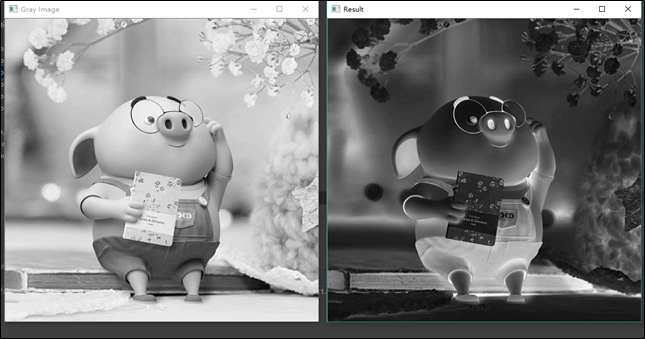3 非线性灰度变换

3.1 对数变换

图像灰度对数变换一般表示如下所示：

其中，表示原始图像的灰度级，表示变换后的灰度级，为常数。

假设，下图所示的对数曲线的形状表明，改变换将输入中范围较窄的低灰度值映射为输出中较宽范围的灰度值。相反的，对高的输入灰度值也是如此。我们使用这种类型的变换来扩展图像中暗像素的值，同时压缩更高灰度级的值。反对数变换的作用与此相反。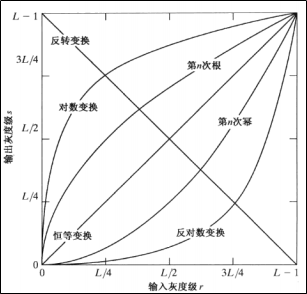代码如下所示：

# -*- coding: utf-8 -*-
import numpy as np
import matplotlib.pyplot as plt
import cv2

#绘制曲线
def log_plot(c):
x = np.arange(0, 256, 0.01)
y = c * np.log(1 + x)
plt.plot(x, y, 'r', linewidth=1)
plt.rcParams['font.sans-serif']=['SimHei'] #正常显示中文标签
plt.title(u'对数变换函数')
plt.xlim(0, 255), plt.ylim(0, 255)
plt.show()

#对数变换
def log(c, img):
output = c * np.log(1.0 + img)
output = np.uint8(output + 0.5)
return output

#读取原始图像

#绘制对数变换曲线
log_plot(42)

#图像灰度对数变换
output = log(42, img)

#显示图像
cv2.imshow('Input', img)
cv2.imshow('Output', output)
cv2.waitKey(0)
cv2.destroyAllWindows()


运行结果如下图所示：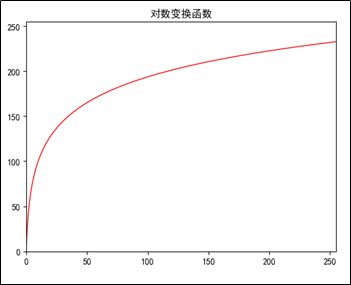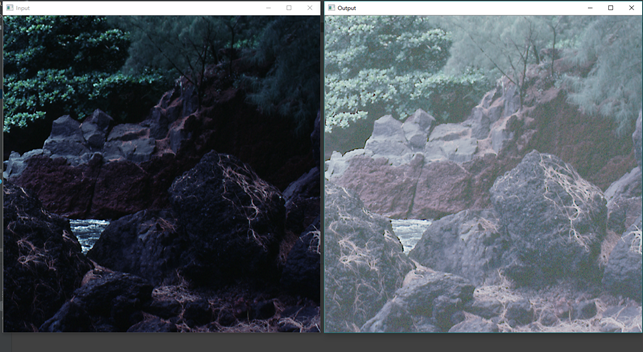3.2 伽马变换

伽玛变换又称为 指数变换 或 幂次变换，是另一种常用的灰度非线性变换。图像灰度的伽玛变换一般表示如下所示：

其中，表示原始图像的灰度级，表示变换后的灰度级，和正常数

1）当>1时，会拉伸图像中灰度级较高的区域，压缩灰度级较低的部分；

2）当<1时，会拉伸图像中灰度级较低的区域，压缩灰度级较高的部分；

3）当=1时，该灰度变换是线性的，此时通过线性方式改变原图像。

如下图所示，不同值的变换曲线：下图所示为图像伽马变换的例子：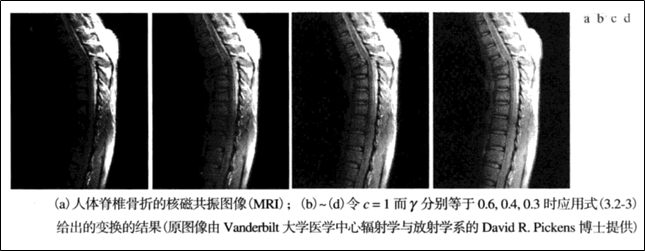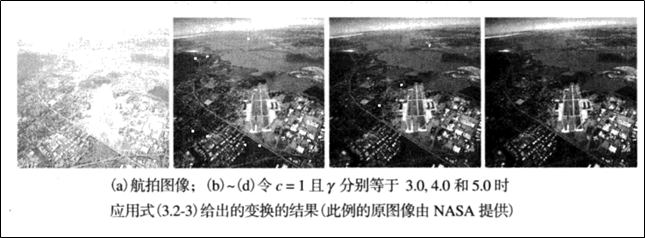代码如下所示：

# -*- coding: utf-8 -*-
import numpy as np
import matplotlib.pyplot as plt
import cv2

#绘制曲线
def gamma_plot(c, v):
x = np.arange(0, 256, 0.01)
y = c*x**v
plt.plot(x, y, 'r', linewidth=1)
plt.rcParams['font.sans-serif']=['SimHei'] #正常显示中文标签
plt.title(u'伽马变换函数')
plt.xlim([0, 255]), plt.ylim([0, 255])
plt.show()

#伽玛变换
def gamma(img, c, v):
lut = np.zeros(256, dtype=np.float32)
for i in range(256):
lut[i] = c * i ** v
output_img = cv2.LUT(img, lut) #像素灰度值的映射
output_img = np.uint8(output_img+0.5)
return output_img

#读取原始图像

#绘制伽玛变换曲线
gamma_plot(0.00000005, 4.0)

#图像灰度伽玛变换
output = gamma(img, 0.00000005, 4.0)

#显示图像
cv2.imshow('Imput', img)
cv2.imshow('Output', output)
cv2.waitKey(0)
cv2.destroyAllWindows()

运行结果如下图所示：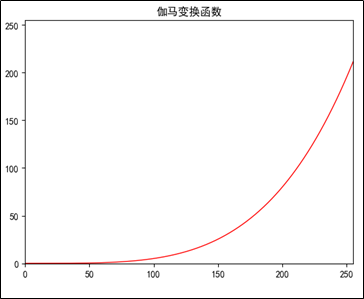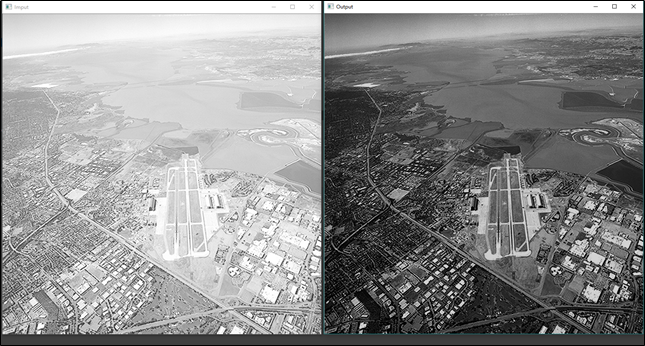参考资料

 https://blog.csdn.net/Eastmount/article/details/88858696

 https://blog.csdn.net/Eastmount/article/details/88929290

 冈萨雷斯. 数字图像处理(第三版)

展开全文数字图像处理 Python OpenCV
1.利用imadjust函数实现图像的变换（应该熟悉此函数）

r=i;
r(:,:,2)=0;%将原图像变成单色图像，保留红色
r(:,:,3)=0;
g=i;
g(:,:,1)=0;%将原图像变成单色图像，保留绿色
g(:,:,3)=0;
b=i;
b(:,:,1)=0;%将原图像变成单色图像，保留蓝色
b(:,:,2)=0;
i1=r1+g1+b1;%求变换后的rgb图像
set(0,‘defaultFigurePosition’,[100,100,1000,500]);%修改图形图像的默认设置
set(0,‘defaultFigureColor’,[1 1 1]);%修改图形背景颜色的设置
figure(1)
subplot(121),imshow®;%绘制图像，观察线性灰度变换结果
subplot(122),imshow(r1);
figure(2)
subplot(121),imshow(g);
subplot(122),imshow(g1);%subplot(m,n,i):%把图形窗口分为m×n个子图,并在第i个子图中画图
figure(3)
subplot(121),imshow(b);
subplot(122),imshow(b1);%subplot(m,n,i):
figure(4)
subplot(121),imshow(i);
subplot(122),imshow(i1);%subplot(m,n,i):

2.将图像存储为数值矩阵，利用for循环，判断语句来调整图像

j=rgb2gray(i);
[m,n]=size(j);%获得灰度图像数据j的行列数
x=1;y=1;
for x=1:m
for y=1:n
if(j(x,y)<=35)%对灰度图像进行分段处理
h(x,y)=j(x,y)10;
elseif(j(x,y)>35&j(x,y)<75)
h(x,y)=(10/7)[j(x,y)-5]+50;
else(j(x,y)>75)
h(x,y)=(105/180)*[j(x,y)-75]+150;
end
end
end
set(0,‘defaultFigurePosition’,[100,100,1000,500]);
set(0,‘defaultFigureColor’,[1 1 1]);
subplot(121),imshow(j);
subplot(122),imshow(h);

3.将图像作为一个整体操作

g=rgb2gray(i);
j=double(g);%数据类型转换为双精度
h=(log(j+1))/10;%基于常用对数的非线性灰度变换
set(0,‘defaultFigurePosition’,[100,100,1000,500]);
set(0,‘defaultFigureColor’,[1 1 1]);
subplot(121),imshow(g);
subplot(122),imshow(h);


展开全文• 灰度变换，属于一个非常重要的概念。这里主要参考《Digital Image Processing》 Rafael C. Gonzalez / Richard E. Woods 的第三章。书中所有的实验与数学式都采用了8-bit 图像的灰度范围，也就是0到255这样一个范围...
灰度变换，属于一个非常重要的概念。这里主要参考《Digital Image Processing》 Rafael C. Gonzalez / Richard E. Woods 的第三章。书中所有的实验与数学式都采用了8-bit 图像的灰度范围，也就是0到255这样一个范围，这是本书不合理的一个地方。首先，这样做并不泛用，图片不一定是8-bit的。其次，在做某些变换的时候，可能会导致溢出。比如，伽马变化，假设伽马值为2，那么灰度为255的像素点，其变换之后值为65025，这里就溢出了。当然，要是使用Matlab计算，肯定会处理的非常好，直接使用mat2gray函数就能将其压缩回0到255。但是要是其他嵌入式平台处理的时候，直接套用不方便不说，直接按照8-bit的图来理解很不直观。因此，我将数学式做了改变，让其输入为0到1的浮点数，其输出也是0到1的浮点数，这样方便理解。

本文所使用的图片，均来源于《Digital Image Processing》的主页 http://www.imageprocessingplace.com/

图像反转

图像反转，这个翻译还是很不恰当的。这里应该理解为负片变换，负片变换如下所示。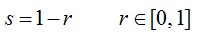负片变换，主要用于观察过黑的图片，负片变换之后，方便观察。很简单的变换。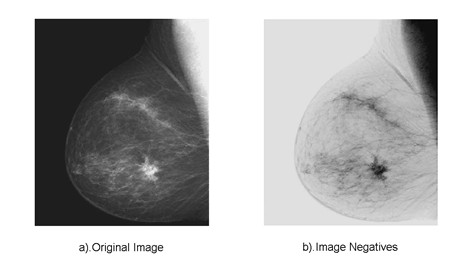对数变换

对数变换主要用于将图像的低灰度值部分扩展，将其高灰度值部分压缩，以达到强调图像低灰度部分的目的。变换方法由下式给出。这里的对数变换，底数为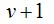，实际计算的时候，需要用换底公式。其输入为，其输出也为。对于不同的底数，其对应的变换曲线如下图所示。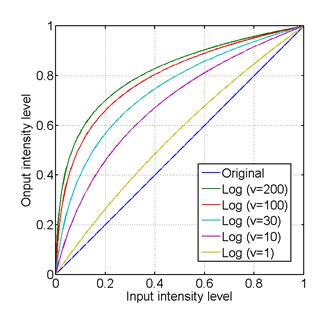底数越大，对低灰度部分的强调就越强，对高灰度部分的压缩也就越强。相反的，如果想强调高灰度部分，则用反对数函数就可以了。看下面的实验就可以很直观的理解，下图是某图像的二维傅里叶变换图像，其为了使其灰度部分较为明显，一般都会使用灰度变换处理一下。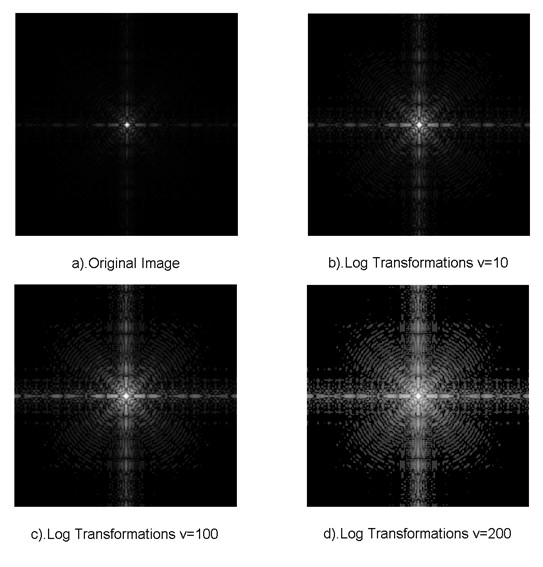实现对数变换的Matlab代码如下：

[plain] view plaincopy

close all;
clear all;

%% -------------Log Transformations-----------------
f = mat2gray(f,[0 255]);

v = 10;
g_1 = log2(1 + v*f)/log2(v+1);

v = 30;
g_2 = log2(1 + v*f)/log2(v+1);

v = 200;
g_3 = log2(1 + v*f)/log2(v+1);

figure();
subplot(1,2,1);
imshow(f,[0 1]);
xlabel('a).Original Image');
subplot(1,2,2);
imshow(g_1,[0 1]);
xlabel('b).Log Transformations v=10');

figure();
subplot(1,2,1);
imshow(g_2,[0 1]);
xlabel('c).Log Transformations v=100');

subplot(1,2,2);
imshow(g_3,[0 1]);
xlabel('d).Log Transformations v=200');
伽马变换

伽马变换主要用于图像的校正，将漂白的图片或者是过黑的图片，进行修正。伽马变换也常常用于显示屏的校正，这是一个非常常用的变换。其变化所用数学式如下所示，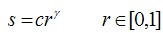其输入为，其输出也为。对于不同的伽马值，其对应的变换曲线如下图所示。和对数变换一样，伽马变换可以强调图像的某个部分。根据下面两个实验，可以看出伽马变换的作用。

实验1：其实现Matlab代码为：

[plain] view plaincopy

close all;
clear all;

%% -------------Gamma Transformations-----------------
f = mat2gray(f,[0 255]);

C = 1;
Gamma = 0.4;
g2 = C*(f.^Gamma);

figure();
subplot(1,2,1);
imshow(f,[0 1]);
xlabel('a).Original Image');

subplot(1,2,2);
imshow(g2,[0 1]);
xlabel('b).Gamma Transformations \gamma = 0.4');
实验2：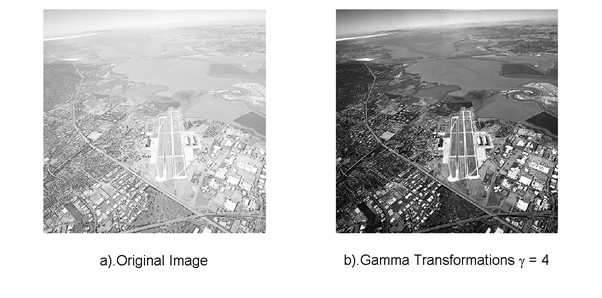灰度拉伸

灰度拉伸也用于强调图像的某个部分，与伽马变换与对数变换不同的是，灰度拉升可以改善图像的动态范围。可以将原来低对比度的图像拉伸为高对比度图像。实现灰度拉升的方法很多，其中最简单的一种就是线性拉伸。而这里介绍的方法稍微复杂一些。灰度拉伸所用数学式如下所示。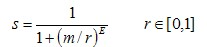同样的，其输入为，其输出也为。这个式子再熟悉不过了，跟巴特沃斯高通滤波器像极了，其输入输出关系也大致能猜到是个什么形状的。但是，这里就出现一个问题了，输入为0时候，式子无意义了。所以，在用Matlab计算的时候，将其变为如下形式。这里的eps，就是Matlab里面，一个很小数。如此做的话，式子变得有意义了。但是，其输入范围为的时候，其输出范围变为了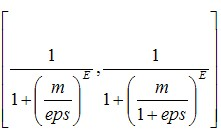。输出范围大致为，为了精确起见，使用mat2gray函数将其扩展到精确的。调用格式如下。

[plain] view plaincopy

g = mat2gray(g,[1/(1+(m/eps)^E) 1/(1+(m/1+eps)^E)]);
输入输出问题解决了，还有一个问题，参数的决定。这里有两个参数，一个是m（相对于巴特沃斯高通滤波器而言，这个是截止频率），一个是E（相对于巴特沃斯高通滤波器而言，这个是滤波器次数）。m可以控制变换曲线的重心，E则可以控制曲线的斜率，如下图所示。m值的可取图像灰度分布的中央值，如下式所示，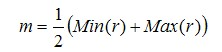决定m之后，接下来就只剩E了。灰度拉升的目的就是扩展图片的动态范围，我们想将原本灰度范围是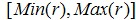的图像变换到内。那么，就直接取最大值与最小值，带入式子，解出E就可以了。但是，如之前所说的，我们所用的式子的的输出范围达不到，而且，直接取的范围，会造成E非常大，从而变换曲线的斜率非常大，灰度扩展的结果并不是很好。所以，这里退一步，取的输出范围是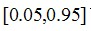。E的取值，如下所示。实验：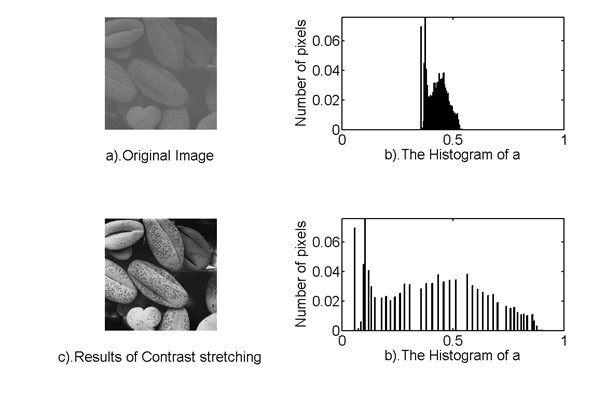从直方图看，原图的灰度范围确实被拉伸了。用上面所说的方法，确定的灰度拉伸的输入输出曲线如下图所示。其Matlab代码如下：

[plain] view plaincopy

close all;
clear all;

%% -------------Contrast Stretching-----------------
f = mat2gray(f,[0 255]);

[M,N] = size(f);
g = zeros(M,N);

Min_f = min(min(f));
Max_f = max(max(f));
m = (Min_f + Max_f)/2;

Out_put_min = 0.05;
Out_put_max = 0.95;

E_1 = log(1/Out_put_min - 1)/log(m/(Min_f+eps));
E_2 = log(1/Out_put_max - 1)/log(m/(Max_f+eps));
E = ceil(min(E_1,E_2)-1);

g = 1 ./(1 + (m ./ (f+ eps)).^E);
g = mat2gray(g,[1/(1+(m/eps)^E) 1/(1+(m/1+eps)^E)]);

figure();
subplot(2,2,1);
imshow(f,[0 1]);
xlabel('a).Original Image');

subplot(2,2,2);
r = imhist(f)/(M*N);
bar(0:1/255:1,r);
axis([0 1 0 max(r)]);
xlabel('b).The Histogram of a');
ylabel('Number of pixels');

subplot(2,2,3);
imshow(g,[0 1]);
xlabel('c).Results of Contrast stretching');

subplot(2,2,4);
s = imhist(g)/(M*N);
bar(0:1/255:1,s);
axis([0 1 0 max(s)]);
xlabel('b).The Histogram of a');
ylabel('Number of pixels');

in_put = 0:1/255:1;
Out_put1 = 1 ./(1 + (m ./ (double(in_put)+ eps)).^E);
Out_put1 = mat2gray(Out_put1,[1/(1+(m/eps)^E) 1/(1+(m/1+eps)^E)]);

figure();
plot(in_put,Out_put1);
axis([0,1,0,1]),grid;
axis square;
xlabel('Input intensity level');
ylabel('Onput intensity level');
灰度切割

灰度切割也是一个很简单，但也很实用的变换。灰度切割，主要用于强调图像的某一部份，将这个部分赋为一个较高的灰度值，其变换对应关系如下所示。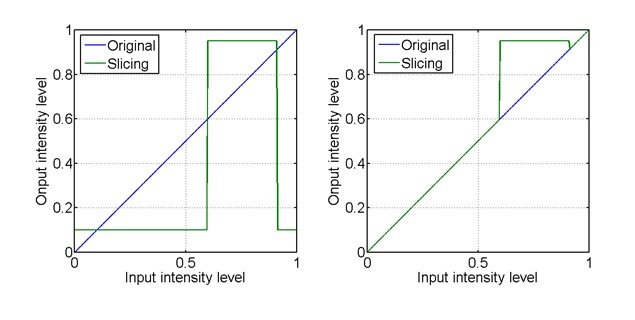灰度切割有以上两种方法，一种是特定灰度值的部分赋值为一个较高的灰度值，其余部分为一个较低的灰度值。这样的方法，得到的结果是一个二值化图像。另外一种方法，则是仅仅强调部分赋值为一个较高的灰度值，其余的部分不变。

实验：位图切割

位图切割，就是按照图像的位，将图像分层处理。若图像的某个像素，其bit7为1，则在位面7这个像素值为1，反之则为0。

实验：由位图切割的结果，图像的主要信息包含在了高4位。仅仅靠高4位，还原的图像更原图基本差不多。由此可见，位图切割主要用于图像压缩。
展开全文• 图像的灰度线性变换是图像灰度变换的一种，图像的灰度变换通过建立灰度映射来调整源图像的灰度，从而达到图像增强的目的。灰度映射通常是用灰度变换曲线来进行表示。通常来说，它是将图像的像素值通过指定的线性函数...灰度变换与空间滤波
• 灰度变换基本函数 工作需要，最近在学习数字图像处理，权当就是一个记录了，把书上的例子自己实现一下加深理解，代码若有问题也欢迎大佬指定。 会根据自己的学习进度一点点进行更新，最理想情况当然是把学到的知识点...matlab
• 1. 图像反转灰度级范围[0, L - 1]的一幅图像的反转图像可以由下式给出：s = L -1 -r .图像反转可以用于处理特别适用于增强嵌入在一幅图像的暗区域中的白色或灰色细节，特别是当黑色面积在尺寸上占主导地位时。 如：...matlab 数字图像处理
• 点操作的增强方法也叫灰度变换。 方法一、直接灰度变换 1、灰度求反：对图像求反是将原图灰度值翻转（黑变白，白变黑，普通黑白底片和照片的关系）。 2、增强对比度：增强图像对比度实际是增强原图的各部分的反差...
• 灰度分为256阶，用灰度表示的图像称作灰度图.在图像中用0~255表示，0是全黑，255是全白 2.对比度：对比度值一幅图像中敏感区域最亮的白和最暗的黑之间的不同亮度层级的测量，差异范围越大代表对比月大。好的对比率...matlab 对比度
• 前两节都是熟悉一下怎么在matlab底下对图片做一些操作，并没有什么卵用，这一节稍微有点卵用，灰度变换一般是图像处理的第一步。 数字图像处理实验1-9点击下列链接有源码和链接： matlab数字图像处理实验 ...matlab 数字图像处理 灰度变换
• 前一篇文章讲解了图像灰度化处理及线性变换知识，结合OpenCV调用cv2.cvtColor()函数实现图像灰度操作，本篇文章主要讲解非线性变换，使用自定义方法对图像进行灰度化处理，包括对数变换和伽马变换。本文主要讲解灰度...Python图像处理 灰度非线性变换 对数变换
• 对数变换可以将图像的低灰度值部分扩展，显示出低灰度部分更多的细节，将其高灰度值部分压缩，减少高灰度值部分的细节，从而达到强调图像低灰度部分的目的； 伽马变换主要用于图像的校正，将灰度过高或者灰度过低的...对数变换 幂律变换 灰度变换
• 通过灰度变换函数，调整输入低质图像的灰度值范围。将图像的低灰度值部分扩展，高灰度值部分压缩。借此强调图像低灰度部分，达到增强图像的目的。 该法用于图像增强的原理： 显示器无法显示大范围灰度值时，许多...Matlab log
• 灰度变换，及按照一定规则对像素点的灰度值进行变换，变换的结果可以增强对比度，或者达到其他的效果（例如二值化，或者伽马变换），由于灰度变换为针对单个像素点的灰度值进行变换，素以算法复杂度一般为O（W*H）...图像处理 opencv 灰度变换
• ## 灰度线性变换

千次阅读 2015-09-28 13:22:08
以灰度图像为例，假设原图像像素的灰度值为D = f(x,y), (x,y)为图像坐标，处理后图像像素的灰度值为D’ = g(x,y),则灰度变换函数可以表示为： g(x,y) = T[f(x,y)] 或 D = T[D] 要求D和D’都在图像的灰度范围之内。...
• ## MATLAB的图像灰度线性变换

万次阅读 多人点赞 2018-11-19 10:03:42MATLAB 灰度处理 线性变换图像处理 matlab 线性变换 直方图均衡化MATLAB 图像增强 灰度变换 图像处理